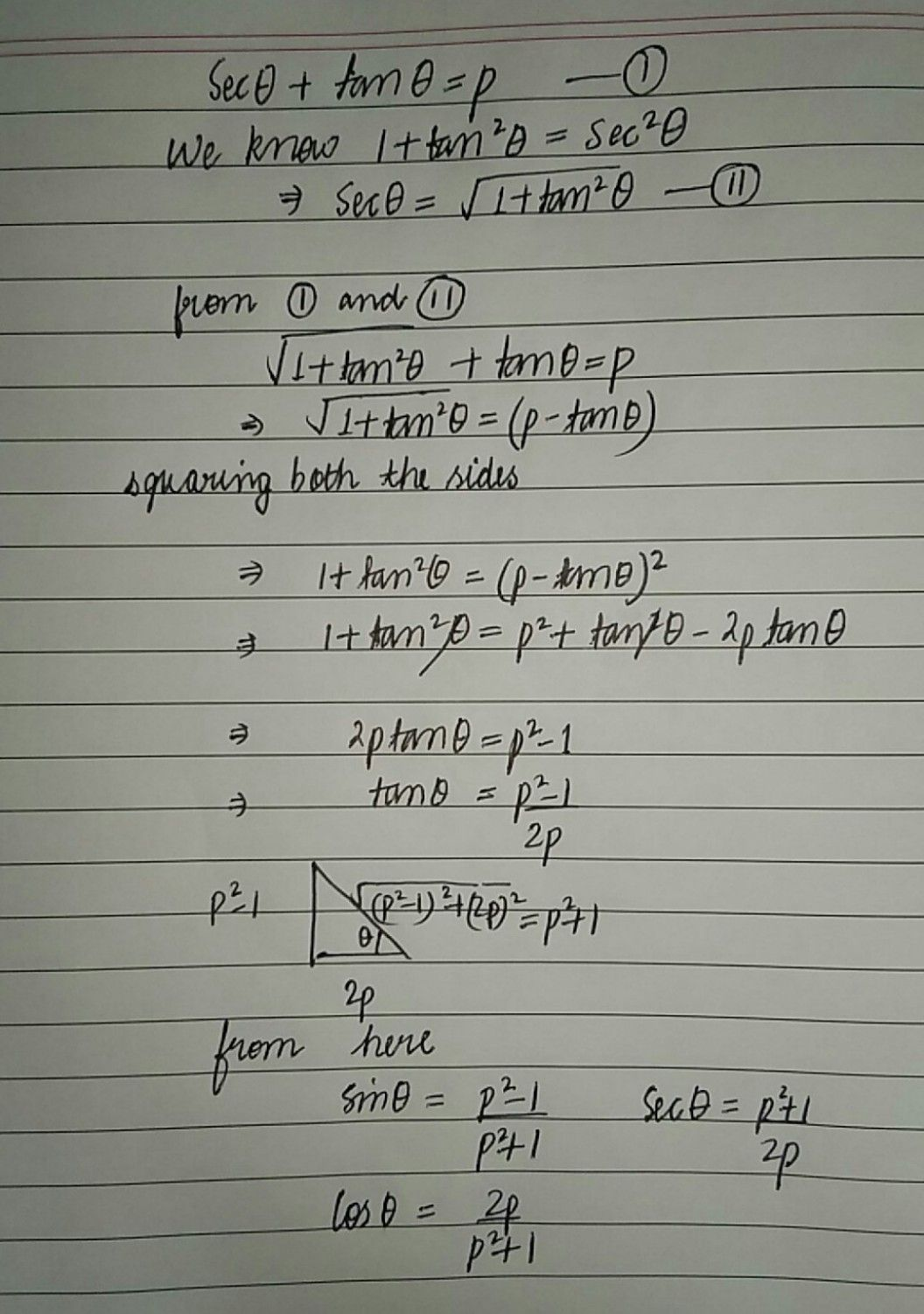Symbol
Problemif sec theta $-$ $-610$ $l7=1s=O\right)$ then find the sin theta , tan theta , sec they value of $9$ terms of p.
Algebra
SolutionQanda teacher - priyanka